How to Calculate the Acid Ionization Constant

Instructor: Edward Kabara

Edward is an adjunct professor in several colleges across Michigan and has a Ph.D. in Biochemistry.

In this lesson we will describe the acid ionization constant. Using basic equations we will calculate the constant first. Next we use it to compare the relative strengths of different acids. Also, we cover how to calculate the constant for acids that have multiple protons that could be lost in a solution.

Introduction to the Acid Ionization ConstantWhen you wake up in the morning, you might start the day with a nice glass of orange juice. The delicious acid tang hits your tongue, and you know today is going to go well. But then you may find yourself wondering: why is it safe to drink orange juices while drinking a similar amount of hydrochloric acid would probably not end as well? The answer lies in the acid ionization constant. In this lesson, we will calculate the acid ionization constant, describe its use, and use it to understand how different acids have different strengths.

Definition of the Acid Ionization Constant

The acid ionization constant, also called the 'acid dissociation constant' or 'acidity constant,' is a measure of how much of the acid is deprotonated (which is when it's unbound to a hydrogen), compared to molecules still bound to a hydrogen. Let's look at a simple example of an acid: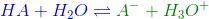In this example the acid HA becomes deprotonated by losing a hydrogen to the water and becoming A-. We know that not all acid HA will become deprotonated, so we can write an constant to establish a ratio of how much has and has not reacted with the water. Much like any other chemical equilibrium formula, we can write the equilibrium constant as: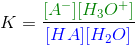Remember that a molecule in brackets such as this one...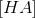...is the molar concentration of the molecule, the number of moles of HA per liter of solution in this case water. We're multiplying the molar concentration of the products, chemicals to the right of the equation, and dividing this by the concentration of the reactants, chemicals to the left of the equation. But, if we use the K above, this will almost always be an extremely small number because most of a water solution is water. Thus, we can modify the above equilibrium constant to this: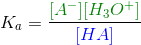We are no longer looking at the amount of water in the solution, and the K changes from a standard equilibrium constant to a much more manageable value and becomes Ka, the acid ionization constant.

Real Example of an Acid Ionization Constant

As an example, let's look at hydrochloric acid, HCl. When we add solid HCl to water, we have the following reaction: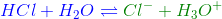We then complete the equilibrium constant like we did above by dividing the concentration of the products by the reactants:

Next, we remove the concentra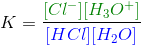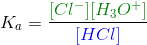To unlock this lesson you must be a Study.com Member.

Register to view this lesson

Are you a student or a teacher?